JEE  >  Test: Comprehension Based Questions: Circle

# Test: Comprehension Based Questions: Circle

Test Description

## 8 Questions MCQ Test Maths 35 Years JEE Main & Advanced Past year Papers | Test: Comprehension Based Questions: Circle

Test: Comprehension Based Questions: Circle for JEE 2023 is part of Maths 35 Years JEE Main & Advanced Past year Papers preparation. The Test: Comprehension Based Questions: Circle questions and answers have been prepared according to the JEE exam syllabus.The Test: Comprehension Based Questions: Circle MCQs are made for JEE 2023 Exam. Find important definitions, questions, notes, meanings, examples, exercises, MCQs and online tests for Test: Comprehension Based Questions: Circle below.
Solutions of Test: Comprehension Based Questions: Circle questions in English are available as part of our Maths 35 Years JEE Main & Advanced Past year Papers for JEE & Test: Comprehension Based Questions: Circle solutions in Hindi for Maths 35 Years JEE Main & Advanced Past year Papers course. Download more important topics, notes, lectures and mock test series for JEE Exam by signing up for free. Attempt Test: Comprehension Based Questions: Circle | 8 questions in 10 minutes | Mock test for JEE preparation | Free important questions MCQ to study Maths 35 Years JEE Main & Advanced Past year Papers for JEE Exam | Download free PDF with solutions
 1 Crore+ students have signed up on EduRev. Have you?
Test: Comprehension Based Questions: Circle - Question 1

### PASSAGE-1 ABCD is a square of side length 2 units. C1 is the circle touching all the sides of the square ABCD and C2 is the circumcircle of square ABCD. L is a fixed line in the same plane and R is a fixed point. Q. If P is any point of C1 and Q is another point on C2, then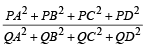is equal to (2006 - 5M, –2)

Detailed Solution for Test: Comprehension Based Questions: Circle - Question 1

Without loss of generality we can assume the square ABCD with its vertices A (1, 1), B  (–1, 1), C (–1, –1), D (1, –1)
P to be the point (0, 1) and Q as (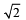,0 ).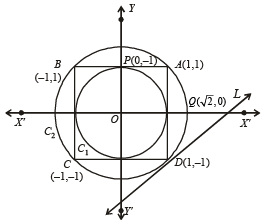Then,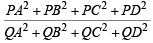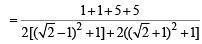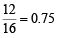Test: Comprehension Based Questions: Circle - Question 2

### PASSAGE-1 ABCD is a square of side length 2 units. C1 is the circle touching all the sides of the square ABCD and C2 is the circumcircle of square ABCD. L is a fixed line in the same plane and R is a fixed point. Q. If a circle is such that it touches the line L and the circle C1 externally, such that both the circles are on the same side of the line, then the locus of centre of the circle is (2006 - 5M, –2)

Detailed Solution for Test: Comprehension Based Questions: Circle - Question 2

Let C' be the said circle touching C1 and L, so that C1 and C' are on the same side of L. Let us draw a line T parallel to L at a distance equal to the radius of circle C1, on opposite side of L.
Then the centre of C' is equidistant from the centre of C1 and from line T.
⇒ locus of centre of C' is a parabola.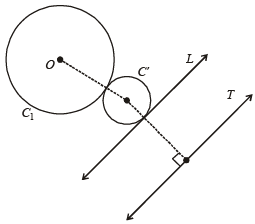Test: Comprehension Based Questions: Circle - Question 3

### PASSAGE-1 ABCD is a square of side length 2 units. C1 is the circle touching all the sides of the square ABCD and C2 is the circumcircle of square ABCD. L is a fixed line in the same plane and R is a fixed point. Q. A line L' through A is drawn parallel to BD. Point S moves such that its distances from the line BD and the vertex A are equal. If locus of S cuts L' at T2 and T3 and AC at T1, then area of ΔT1T2T3 is (2006 - 5M, –2)

Detailed Solution for Test: Comprehension Based Questions: Circle - Question 3

Since S is equidistant form A and line BD, it traces a parabola. Clearly, AC is the axis, A (1, 1) is the focus

and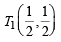is the vertex of parabola.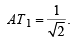T2 T3 = latus rectum of parabola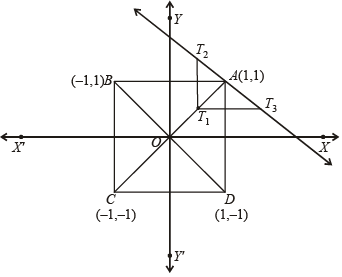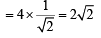∴ Area (ΔT1T2T3) =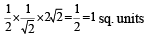Test: Comprehension Based Questions: Circle - Question 4

PASSAGE-2
A circle C of radius 1 is inscribed in an equilateral triangle PQR.
The points of contact of C with the sides PQ, QR, RP are D, E, F, respectively. The line PQ is given by the equation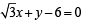and the point D is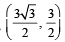Further, it is given that the origin and the centre of C are on the same side of the line PQ.

Q. The equation of circle C is (2008)

Detailed Solution for Test: Comprehension Based Questions: Circle - Question 4

Slope of CD =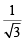∴ Parametric equation of CD is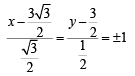∴ Two possible coordinates of C are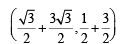or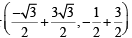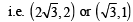As (0, 0) and C lie on the same side of PQ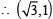should be the coordinates of C.
NOTE THIS STEP :  Remember (x1, y1) and (x2, y2) lie on the same or opposite side of a line ax + by + c = 0 according as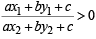∴ Equation of the circle is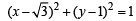Test: Comprehension Based Questions: Circle - Question 5

PASSAGE-2
A circle C of radius 1 is inscribed in an equilateral triangle PQR.
The points of contact of C with the sides PQ, QR, RP are D, E, F, respectively. The line PQ is given by the equationand the point D isFurther, it is given that the origin and the centre of C are on the same side of the line PQ.

Q. Points E and F are given by (2008)

Detailed Solution for Test: Comprehension Based Questions: Circle - Question 5

ΔPQR is an equilateral triangle, the incentre  C must coincide with centriod of ΔPQR and D, E, F must concide with the mid points of sides PQ, QR and RP respectively.

Also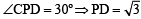Writing the equation of side PQ in symmetric form we

get,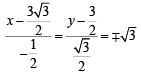∴ Coordinates of P =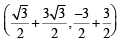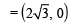and

coordinates of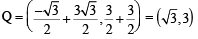Let coordinates of R be (α,β) , then using the formula for centriod of Δ we get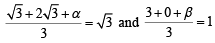⇒ α = 0 and β = 0

∴ Coordinates of R = (0, 0)

Now coordinates of E = mid point of QR  =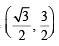and coordinates of F = mid point of PR =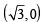Test: Comprehension Based Questions: Circle - Question 6

PASSAGE-2
A circle C of radius 1 is inscribed in an equilateral triangle PQR.
The points of contact of C with the sides PQ, QR, RP are D, E, F, respectively. The line PQ is given by the equationand the point D isFurther, it is given that the origin and the centre of C are on the same side of the line PQ.

Q. Equations of the sides QR, RP are (2008)

Detailed Solution for Test: Comprehension Based Questions: Circle - Question 6

Equation of side QR is y =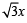and equation of side RP is  y = 0

Paragraph 3 Given the implicit function y3 – 3y + x = 0

For x ∈(–∞, –2) ∪ (2,∞) it is y = f (x) real valued differentiable function and for x ∈ (–2, 2) it is y = g(x) real valued differentiable function.

Test: Comprehension Based Questions: Circle - Question 7

PASSAGE-3
A tangent PT is drawn to the circle x2 + y2 = 4 at the point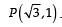. A straight line L, perpendicular to PT is a tangent to the circle (x – 3)2 + y2 = 1.    (2012)

Q. A possible equation of L is

Detailed Solution for Test: Comprehension Based Questions: Circle - Question 7

Equation of tangent PT to the circle x2 + y2 = 4 at the point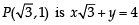Let the line L, perpendicular to tangent PT be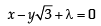As it is tangent to the circle (x – 3)2 + y2 = 1

∴ length of perpendicular from centre of circle to the tangent = radius of circle.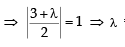= – 1 or  – 5

∴ Equation of L can be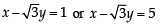Test: Comprehension Based Questions: Circle - Question 8

PASSAGE-3
A tangent PT is drawn to the circle x2 + y2 = 4 at the point. A straight line L, perpendicular to PT is a tangent to the circle (x – 3)2 + y2 = 1.    (2012)

Q. A common tangent of the two circles is

Detailed Solution for Test: Comprehension Based Questions: Circle - Question 8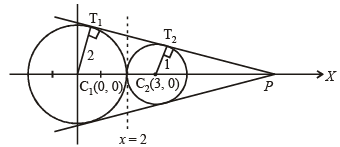From the figure it is clear that the intersection point of two direct common tangents lies on x-axis.
Also ΔPT1C1 ~ ΔPT2C2 ⇒ PC1 : PC2 = 2 : 1
or P divides C1C2 in the ratio 2 : 1 externally
∴ Coordinates of P are (6, 0) Let the equation of tangent through P be y = m (x – 6)
As it touches x2 + y2 = 4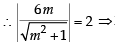36 m2 = 4(m2 + 1)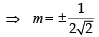∴ Equations of common tangents are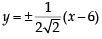Also x = 2 is the common tangent to the two circles.

## Maths 35 Years JEE Main & Advanced Past year Papers

132 docs|70 tests
 Use Code STAYHOME200 and get INR 200 additional OFF Use Coupon Code
Information about Test: Comprehension Based Questions: Circle Page
In this test you can find the Exam questions for Test: Comprehension Based Questions: Circle solved & explained in the simplest way possible. Besides giving Questions and answers for Test: Comprehension Based Questions: Circle, EduRev gives you an ample number of Online tests for practice

## Maths 35 Years JEE Main & Advanced Past year Papers

132 docs|70 tests